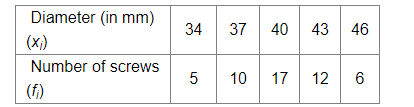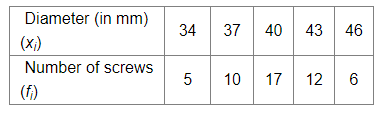# The measurements (in mm) of the diameters of the heads of 50 screws are given below:

Question:

The measurements (in mm) of the diameters of the heads of 50 screws are given below:Calculate the mean diameter of the heads of the screws.

Solution:

We know that,

Mean $=\frac{\sum x_{i} f_{i}}{\sum f_{i}}$

For the following data:Mean $=\frac{(34 \times 5)+(37 \times 10)+(40 \times 17)+(43 \times 12)+(46 \times 6)}{5+10+17+12+6}$

$=\frac{170+370+680+516+276}{50}$

$=\frac{2012}{50}$

$=40.24$

Hence, the mean diameter of the heads of the screws is 40.24 .